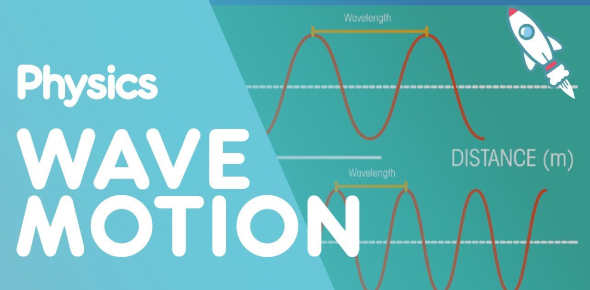# Trivia Questions On Waves! Quiz

32 Questions | Total Attempts: 647SettingsA wave can be described as a disturbance that travels through a medium from one location to another. A wave is the propagation of disturbances from place to place in a regular and organized way. The most familiar are surface waves that travel on water. If you want to become an expert about waves, complete this quiz.

• 1.
The energy of a wave is directly related to its
• A.

Amplitude.

• B.

Wavelength.

• C.

Frequency.

• D.

Speed.

• 2.
The substance through which a wave travels is called a
• A.

Source.

• B.

Trough.

• C.

Disturbance.

• D.

Medium.

• 3.
The seismic waves that travel the fastest are
• A.

Surface waves.

• B.

S waves.

• C.

P waves.

• D.

Tsunamis.

• 4.
All waves with a frequency of 100 hertz.
• A.

Travel 100 meters in one second.

• B.

Measure 100 meters from crest to crest.

• C.

Complete 100 vibrations in one second.

• D.

Measure 100 meters from crest to trough.

• 5.
A wave that moves a medium at right angles to the direction in which the wave travels is a
• A.

Longitudinal wave.

• B.

Mechanical wave.

• C.

Transverse wave.

• D.

Seismic wave.

• 6.
To increase the amplitude of vibrations, you can adjust the frequency to
• A.

Match the natural resonant frequency.

• B.

Be greater than the natural resonant frequency.

• C.

Be less than the natural resonant frequency.

• D.

Cancel the natural resonant frequency.

• 7.
Which of the following accurately describes surface waves?
• A.

Surface waves are a combination of a longitudinal wave and transverse wave.

• B.

Surface waves move more slowly than P waves and S waves.

• C.

Surface waves can cause the most severe ground movements.

• D.

All the above.

• 8.
The bending of waves around the edge of a barrier is called
• A.

Diffraction.

• B.

Refraction.

• C.

Interference.

• D.

Reflection.

• 9.
The maximum distance the particles of a medium move from their rest position is called the
• A.

Wavelength.

• B.

Amplitude.

• C.

Frequency.

• D.

Speed.

• 10.
Tsunamis are produced by
• A.

Distant typhoon winds.

• B.

Undersea earthquakes.

• C.

Earthquakes that occur only on land.

• D.

Erratic tides.

• 11.
One way in which P waves differ from S waves is that
• A.

S waves travel through Earth's core while P waves do not.

• B.

P waves travel through Earth's core while S waves do not.

• C.

S waves can travel through liquids while P waves cannot.

• D.

P waves are transverse waves while S waves are longitudinal.

• 12.
The combining of two waves to make a wave with a smaller amplitude is called
• A.

A standing wave.

• B.

Resonance.

• C.

Destructive interference.

• D.

Constructive interference.

• 13.
A wave that moves particles of a medium parallel to the direction in which the wave travels is a
• A.

Longitudinal wave.

• B.

Mechanical wave.

• C.

Seismic wave.

• D.

Transverse wave.

• 14.
Which of the following accurately describes surface waves?
• A.

Surface waves are a combination of a longitudinal wave and transverse wave.

• B.

Surface waves move more slowly than P waves and S waves.

• C.

Surface waves can cause the most severe ground movements.

• D.

All of the above.

• 15.
The speed of waves
• A.

Is the same in every medium.

• B.

Is constant in a given medium under uniform conditions.

• C.

Always varies from point to point in a given medium.

• D.

Changes if the frequency or wavelength changes.

• 16.
A wave that appears to be still in one place, even though it is really two waves interfering as they pass through each other is called a(n)
• A.

Longitudinal wave.

• B.

P wave.

• C.

Standing wave.

• D.

S wave.

• 17.
The energy of a wave is directly related to its
• A.

Amplitude.

• B.

Wavelength.

• C.

Frequency.

• D.

Speed.

• 18.
To look for resources deep underground, geologists produce a small explosion at the surface so that they can
• A.

Detect seismic waves reflected off underground structures.

• B.

Uncover hidden structures.

• C.

Detect seismic waves reflected off the surface of the ground.

• D.

Shake up the ground and make cracks for oil to seep upward.

• 19.
The interaction between waves that meet is called
• A.

Diffraction.

• B.

Refraction.

• C.

Interference.

• D.

Reflection.

• 20.
Waves that require a medium through which to travel are called
• A.

Longitudinal waves.

• B.

Mechanical waves.

• C.

Transverse waves.

• D.

Seismic waves.

• 21.
The seismic waves that travel the fastest are
• A.

Surface waves.

• B.

S waves.

• C.

P waves.

• D.

Tsunamis.

• 22.
Seismic waves that travel through Earth's core are
• A.

Surface waves.

• B.

P waves.

• C.

S waves.

• D.

Tsunamis.

• 23.
A wave moving from one medium to another at an angle results in
• A.

Diffraction.

• B.

Refraction.

• C.

Interference.

• D.

Reflection.

• 24.
Transverse waves have
• A.

Crests and troughs.

• B.

Compressions and rarefactions.

• C.

Crest and rarefactions.

• D.

Compressions and troughs.

• 25.
Longitudinal waves have
• A.

Crest and troughs.

• B.

Compressions and rarefactions.

• C.

Compressions and troughs.

• D.

Rarefaction and crests.

Related TopicsBack to top